# NCERT Solutions for Class 8 Maths Chapter 12 Exponents and Powers| PDF Download

Here you will find NCERT Solutions for Class 8 Maths Chapter 12 Exponents and Powers that will be helpful in developing necessary skill to solve more and more questions. You can download PDF of Class 8 Maths Chapter 12 Exponents and Powers NCERT Solutions make you able to solve the difficult problems in a exercise. Through the help of these NCERT Solutions you'll be able to complete your homework on time.

Chapter 12 NCERT Solutions of Class 8 Maths will be useful in understanding the basic concepts and will tell you about the difficulty of questions. These NCERT Solutions will serve as beneficial tool that can be used to recall various questions any time.Page No: 197

Exercise 12.1

1. Evaluate:
(i) 3-2
(ii) (-4)-2
(iii) (1/2)-5

(i) 3-2
= 1/32 [ ∵ a-m = 1/am ]
= 1/9

(ii) (-4)-2
= 1/(-4)2 [∵ a-m = 1/am ]
= 1/16

(iii) (1/2)-5
= (2/1)5  [ ∵ a-m = 1/am ]
= (2)5 = 32

2. Simplify and express the result in power notation with positive exponent:
(i) ( -4)5 ÷ (-4)8
(ii) (1/23)2
(iii) (-3)4 × (5/3)4
(iv) (3-5 ÷ 3-10) × 3-5
(v) 2-3 × (-7)-3

(i) ( -4)5 ÷ (-4)8
= (-4)5-8 [ ∵ am ÷ an = am-n]
= (-4)-3
= 1/(-4)3 [∵ a-m = 1/am ]

(ii) (1/23 )2
= 12/(23)2 [∵ (a/b)m = am/an]
= 1/23×2
= 1/26 [(am)n = am×n]

(iii) (-3)4 × (5/3)4
= (-3)4 × 54/34 [∵ (a/b)m = am/an]
= {(-1)4 × 34} × 54/3[ ∵ (ab)m = ambm]
= 34-4 × 54 [ ∵ am ÷ an = am-n]
= 30 × 54
= 54 [a0 = 1]

(iv) (3-7 ÷ 3-10) × 3-5
= 3-7-(-10) × 3-5 [∵ am ÷ an = am-n]
= 3-7+10 × 3-5
= 33 × 3-5 = 33+(-5) [∵ am × an = am+n]
=3-2
= 1/32 [a-m = 1/am]

(v) 2-3 × (-7)-3
= 1/23 ×1/(-7)3 [∵ a-m = 1/am]
= 1/{2 × (-7)}3
= 1/(-14)3 [∵ (ab)m = ambm]

3. Find the value of:
(i) (30 + 4-1) × 22
(ii) (2-1 × 4-1) ÷ 2-2
(iii) (1/2)-2 + (1/3)-2 + (1/4)-2
(iv) (3-1 + 4-1 + 5-1)0
(v) {(-2/3)-2}2

(i) ( 30 + 4-1) × 22
= (1 + 1/4) × 22 [∵ a-m = 1/am]
= {(4 + 1)/4} × 22
= 5/4 × 22
= 5/22 × 22
= 5 × 22-2 [∵ am ÷ an = am-n]
= 5 × 20
= 5 × 1
= 5 [∵ a0 = 1]
(ii) ( 2-1 × 4-1÷ 2-2 =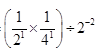[∵ a-m = 1/am][∵ am ×an = am+n]
=[∵ am ÷ an = am-n]
= 1/2 [∵ a-m = 1/am]

(iii) (1/2)-2 + (1/3)-3 + (1/4)-2
= (2-1)-2 + (3-1)-2 + (4-1)-2  [∵ a-m = 1/am]
= 2-1×(-2) + (3)-1×(-2) + (4)-1×(-2) [∵ (am)n = am×n]
= 22 + 32 + 42
= 4 + 9 + 16
= 29

(iv) (3 + 4 + 5)0
= (1/3 + 1/4 + 1/5)0 [∵ a-m = 1/am]
= {(20 + 15 + 12)/60}0
= (47/60)0
= 1 [∵ a0 = 1]

(v)[∵ (am)n = am×n][∵ a-m = 1/am]
= 81/16

4. Evaluate:
(i) (8-1 × 53)/2-4
(ii) ( 5-1 × 2-1) × 6-1

(i) (8-1 × 53)/2-4
= (8-1 × 53)/2-4
= {(23)-1 × 53}/2-4
= (2-3 × 53)/2-4  [∵ (am)n = am×n]
= 2-3(-4) × 53
= 2-3+4 × 53 [∵ am ÷ an = am-n]
= 2×125
= 250

(ii)( 5-1 × 2-1) 6-1
= (1/5 × 1/2) × 1/6 [∵ a-m = 1/am]
= 1/10 × 1/6 = 1/60

5. Find the value of m for which 5m ÷ 5-3 = 55

5m ÷ 5-3 = 55
= 5m-(-3) = 55 [∵ am ÷ an = am-n]
⇒ 5m+3 = 55
Comparing exponents both sides, we get
⇒ m + 3 = 5
⇒ m = 5 - 3
⇒ m = 2

6. Evaluate:
(i)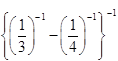(ii)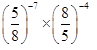(i)=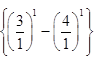[∵ a-m = 1/am]
={3 -4} = -1
(ii)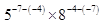[∵ am ÷ an = am-n]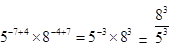[∵ a-m = 1/am]
= 512/125

7. Simplify:
(i)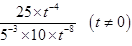(ii)(i)(ii)==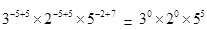= 1×1×3125 [∵ a0 = 1]
= 3125

Page No. 200

Exercise 12.2

1. Express the following numbers in standard form:
(i) 0.0000000000085
(ii) 0.00000000000942
(iii) 6020000000000000
(iv) 0.00000000837
(v) 31860000000

(i) 0.0000000000085
= (0.0000000000085×1012)/1012
= 8.5 × 10-12

(ii) 0.00000000000942
= (0.00000000000942×1012)/1012
= 9.42 ×10-12

(iii) 6020000000000000
= (6020000000000000×1015)/1015
= 6.02 × 1015

(iv) 0.00000000837
= (0.00000000837×109)/109
= 8.37 × 10-9

(v) 31860000000
= 31860000000 × 1010/1010 = 3.186 ×1010

2. Express the following numbers in usual form:
(i) 3.02 × 10-6
(ii) 4.5 × 104
(iii) 3 × 10-8
(iv) 1.0001 × 109
(v) 5.8 × 1012
(vi) 3.61492 × 106

(i) 3.02 × 10-6
= 3.02/106
= 0.00000302

(ii) 4.5 × 104
= 4.5 × 10000
= 45000

(iii) 3 × 10-8
= 3/108
= 0.00000003

(iv) 1.0001 × 109
= 1000100000

(v) 5.8 × 1012
= 5.8 × 1000000000000
= 5800000000000

(vi) 3.61492 × 106
= 3.61492 × 1000000
= 3614920

3. Express the number appearing in the following statements in standard form:
(i)1 micron is equal to 1/1000000 m.
(ii) Charge of an electron is 0.000,000,000,000,000,000,16 coulomb.
(iii) Size of a bacteria is 0.0000005 m.
(iv) Size of a plant cell is 0.00001275 m.
(v) Thickness if a thick paper is 0.07 mm.

(i) 1 micron
= 1/1000000
= 1/106 = 1 × 10-6 m

(ii)Charge of an electron is
0.00000000000000000016 coulombs.
= (0.00000000000000000016×1019)/1019
= 1.6 × 10-19 coulomb

(iii) Size of bacteria = 0.0000005
5/10000000
=5×107
=5×10−7

(iv) Size of a plant cell is 0.00001275 m
= (0.00001275 × 105)/105
= 1.275 × 10-5 m

(v) Thickness of a thick paper = 0.07 mm
= 7/100 mm = 7/102 = 7 × 10-2 mm

4. In a stack there are 5 books each of thickness 20 mm and 5 paper sheets each of thickness 0.016 mm. What is the total thickness of the stack?

Thickness of one book = 20 mm
Thickness of 5 books = 20 × 5 = 100 mm
Thickness of one paper = 0.016 mm
Thickness of 5 papers = 0.016 × 5 = 0.08 mm
Total thickness of a stack = 100 + 0.08
=100.08 mm
= 100.08 × 102/102
= 1.0008 × 102 mm

## NCERT Solutions for Class 8 Maths Chapter 12 Exponents and Powers

Chapter 12 Exponents and Powers will be useful in obtaining good marks in the examinations. 8 × 8 can be written as 82. In 82, the number 8 is called the base and 2 is called the exponent or the power.

• Laws of exponents:
(i) xm × xn = xm+n
(ii) xm ÷ xn = xm-n
(iii) (xm)n = xm×n
(iv) xm × ym = (xy)m
(v) xm/ym = (x/y)m

• The value of any number raised to 0 is 1, i.e. a0 = 1.

• A number is said to be in the standard form, if it is expressed as the product of a number between 1 and 10 and the integral power of 10.

You can find exercisewise NCERT Solutions below that will be help you in finding a specific questions that you're looking for.

Studyrankers subject matter experts have crafter these Class 8 Maths NCERT Solutions by keeping the needs of students in their mind which are accurate and detailed.

### NCERT Solutions for Class 8 Maths Chapters:

 Chapter 1 Rational Numbers Chapter 2 Linear Equations in Variable Chapter 3 Understanding Quadrilaterals Chapter 4 Practical Geometry Chapter 5 Data Handling Chapter 6 Squares and Square Roots Chapter 7 Cubes and Cube Roots Chapter 8 Comparing Quantities Chapter 9 Algebraic Expressions and Identities Chapter 10 Visualising Solid Shapes Chapter 11 Mensuration Chapter 13 Direct and Inverse Proportions Chapter 14 Factorization Chapter 15 Introduction to Graphs Chapter 16 Playing with Numbers

FAQ on Chapter 12 Exponents and Powers

#### How many exercises in Chapter 12 Exponents and Powers Class 8 Maths?

There are total two exercises in the Chapter 12 Class 8 Maths textbook through which a student can learn variety of concepts and formulas that is going to help them in higher classes also. You can clear your doubts if you have any with the help of these NCERT Solutions.

#### If x11 = y0 and x=2y, then y is equal to?

x11 = y0 => x11 = 1 => x = 1. Given, x = 2y hence, y = x/2 =1/2.

63
= 6 × 6 × 6
= 36 × 6
= 216.

#### Write 34500000 in standard form.

34500000 = 345 × 100000
= 3.45 × 100 × 100000
= 3.45 × 102 × 105 = 3.45 × 102+5
= 3.45 × 107
Thus, 34500000 = 3.45 × 107.